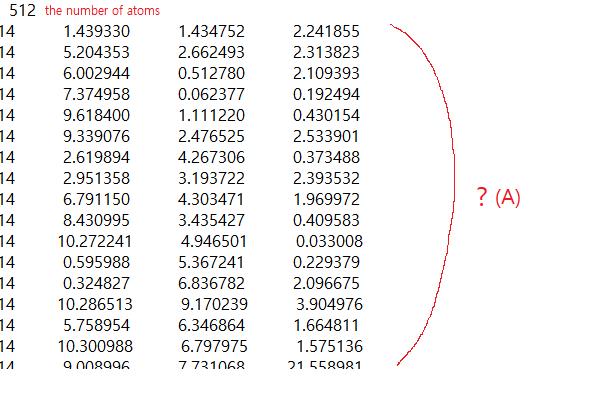# Meaning of eigenvector

Dear. Julian

I have two questions

 in xxx.eig file. like belowas shown in the capture,
For (1) from the second line , are (1) relaxed equilibrium positions of atoms and are (1) same with the atomic position of input?

 is it possible to calculate the displacements of each atom as mode?

 Can I calculate the cartesian or fractional length by converting eigen values using some method?
I know that the the components of x y z of atoms at each mode are the relative size and direction of vibration. is it right? are those component of eigenvector could be converted to dispalcement(u)?
ex u=1/sqrt(M)*eigenvector

Thanks

 The coordinates in the eig file are those for which the phonon calculation was performed. Whether they are optimised or otherwise depends on how you ran the calculation and whether it completed successfully. Likewise they will only be the same as those input if you didn’t optimise.
 The displacement is time-dependent and depends on the eigenvectors. So you can write down the equation for the displacement, but to calculate it you have to assume a time. The average displacement is obviously zero for a harmonic frequency and so there is no overall displacement.
 The eigenvectors are expressed in Cartesian space and diagonalise the dynamical matrix, which means they are already weighted by the inverse mass. To get the displacement would require multiplying by the time-dependent phase factor, allowing for the k point if necessary.

1 Like

In Q3, What is the unit of egenvector (e.g. m , nm , Å)?

The eigenvectors don’t have units - they just transform the dynamical matrix into a set of eigenvalues & so the units are for the eigenvalues (i.e. the squared frequencies).

1 Like

Thanks Julian.

Ok, then do you mean that eigenvalue is sqrt(w^2) ?

The frequencies are the square roots of the eigenvalues of the dynamical matrix.

1 Like

Thank so much julian

I got it!